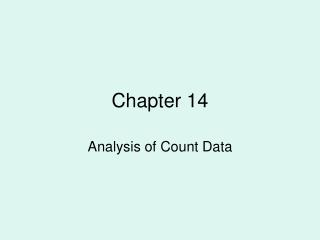DownloadDownload PresentationChapter 14

# Chapter 14

Télécharger la présentation## Chapter 14

- - - - - - - - - - - - - - - - - - - - - - - - - - - E N D - - - - - - - - - - - - - - - - - - - - - - - - - - -
##### Presentation Transcript

1. Chapter 14 Analysis of Count Data

2. Example • You buy a bag of grass seed from Big-K and it produced the following lawn.

3. Example

4. Example • You are concerned because it doesn’t seem to be the same Varity of grasses that were advertised on the bag. You take a representative sample of the lawn to Jeff Houge (CR’s botany instructor) and he gives you the following break down.

5. Example • Annual Ryegrass 1300 pieces • Creeping Red Fescue 525 pieces • Perennial Ryegrass 275 pieces • Other Grasses 25 pieces • Weed & Other 375 pieces

6. Example

7. How To Tell if Significant Difference? • We use the Chi-Squared Goodness-of-fit test. • Data consists of observed counts. • We calculate what we expect to see based on the nulls distribution. Calculate np for each category

8. The Big Idea • 1. The data consist of observedcounts—that is, how many of the items or subjects fall into each category. • 2. We will compute expected counts under Ho, that is, the counts that we would expect to see for each category if the corresponding null hypothesis were true. • 3. We will compare the observed and expected countsto each other via a test statistic that will be a measure of how close the observed counts are to the expected counts under Ho. So if this “distance” is large, we have some support for rejecting Ho.

9. Calculate Chi-Squared

10. Various Chi-Squared Distributions

11. Properties of the Chi-Square Distribution • The distribution is not symmetric and is skewed to the right. • The values are non-negative. • There is a different chi-square distribution for different degrees of freedom. • The mean of the chi-square distribution is equal to its degrees of freedom and is located to the right of the mode. • The variance of the chi-square distribution is 2(df).

12. Do the Test Page 933 • We could calculate the p-value by hand using the TI. To do that we’ll need to know our degrees of freedom which is K – 1 where K is the number of categories. • I wrote a program MULTNOM that is in your calculator to do this test.

13. Let’s Do It • LDI: 14.2, 14.3 • Page 936: Exercises 14.6, 14.10, 14.12, 14.13